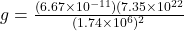## calculate the acceleration due to gravity on the Moon. The Moon’s radius is 1.74×106m1.74×106m and its mass is 7.35×1022kg7.35×1022kg. gMoon

Question

calculate the acceleration due to gravity on the Moon. The Moon’s radius is 1.74×106m1.74×106m and its mass is 7.35×1022kg7.35×1022kg. gMoongMoon = nothing m/s 2 m/s2

in progress 0
2 months 2021-09-01T07:25:35+00:00 1 Answers 29 views 0

Acceleration due to gravity on the surface of moon is given as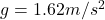Explanation:

As we know that the acceleration due to gravity is given as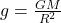now we have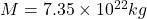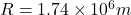now we have